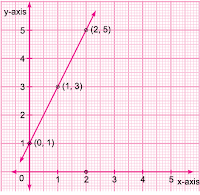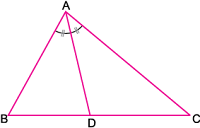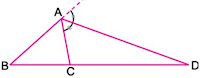## Maths Formula for Class 10

Studyrankers has prepared math formula for CBSE class 10. There are a total of 15 chapters which we have to study in class 10. We have prepared notes from all these chapters. It will help the students in quick revision of all the formulae, identities, theorems in a quick and easy way. Find below the list of all the chapters of class 10 mathematics with their relevant formula.

### Chapter 1 - Real Numbers Formula

Euclid's division lemma
For any two positive integers a and b, there exist (unique) whole numbers q and r such that
a = bq + r, 0≤ r <b.
Euclid's division algorithm is a process based on Euclid's division lemma. According to this, the HCF of two positive integers a and b, with a > b, is obtained as follows:
Step 1: Apply Euclid's division lemma on a and b, a = bq + r, 0 ≤ r <b for some whole numbers q and r.
Step 2: If r = 0, then HCF (a, b) = b.
If r≠0, apply Euclid's division on b and r.
b = rq1 +r1, 0≤ r1 <b for some whole numbers q1 and r1
Step 3: If r1 = 0, then HCF (b,r) = r.
But HCF (a, b) = HCF (b,r) ⇒ HCF (a, b) = r.
Continue the above process until the remainder is zero.
Last non-zero remainder = HCF (a, b)

Fundamental Theorem of Arithmetic
Every composite positive integer n can be expressed (factorised) as a product of primes, and this factorisation is unique, apart from the order in which the prime factors occur.
HCF and LCM of two (or more) natural numbers can be obtained by using Fundamental Theorem of Arithmetic. This method is also called prime factorisation method.
Relationship between HCF and LCM of two natural numbers
HCF × LCM = product of two natural numbers.
LCM of two natural numbers is always divisible by their HCF.

Theorem 1: If a is any natural number and p is a prime number such that p divides a2, then p divides a.
Generalisation of the theorem is:
If a, n are any natural number and p is a prime number such that p divides an, then p divides a.
Theorem 2: If a and b are any natural numbers and p is a prime number such that p divides ab then p divides a or p divides b or p divides both.

Rational and Irrational numbers
A number that can be expressed in the form of p/q, where p, q are integers, q≠0, and p, q are co-prime is called a rational number.
A number that cannot be expressed in the above form is called an irrational number.

Theorem 3: If x is a rational number whose decimal expansion terminates, then x can be expressed as x = p/q where p, q are integers, q≠0 and p, q are co-prime, and the prime factorisation of q is of the form 2m5n where m, n are non-negative integers.

Theorem 4: If x = p/q where p, q are integers, q≠0 and p, q are co-prime such that the prime factorisation of q is of the form 2m5n where m, n are non-negative integers, then x has decimal expansion which terminates.

Theorem 5: If x = p/q where p, q are integers, 90 and p, q are co-prime such that the prime factorisation of q is not of the form 2m5n where m, n are non-negative integers, then x has a decimal expansion which is non-terminating repeating (recurring).
Thus, if x is a rational number and x = p/q where p, q are integers, q≠0 and p, q are co-prime such that the prime factorisation of q has a prime factor other than 2 or 5, then the decimal expansion of x is non-terminating repeating.
The decimal expansion of every rational number is either terminating or non-terminating repeating and conversely every decimal number either with terminating decimal expansion or with non-terminating repeating decimal expansion is a rational number.

### Chapter 2 - Polynomials Formula

Value of a Polynomial: If p(x) is a polynomial and ‘a’ is any real number, then the real number obtained by replacing x by ‘a’ in p(x), is called the value of p(x) at x = a and is represented by p(a).
For example, Let p(x) ≡ x2 + 2x + 5 be a polynomial. The value of p(x) at 3 denoted by p(3) is given by
p(3) = 32 +  2 × 3 + 5 = 20.

Relations between zeroes and coefficients of a polynomial
(i) If a and b are zeroes of the quadratic polynomial ax2 + bx + c, then
α+ β = -b/a and αβ = c/a
(ii) If α, β and γ are zeroes of the cubic polynomial ax3 + bx2 + cx + d, then
α+β+γ = -b/a
αβ + βγ + γα = c/a and αβγ = -d/a.

Division Algorithm for polynomials
If f(x) and g(x) are any two polynomials with g(x)≠0, then there exist (unique) polynomials q(x) and r(x) such that
f(x) = g(x) q(x) + r(x) where,
r(x) = 0 or deg r(x) < deg g(x).
If deg p(x) ≥ deg g(x), then deg q(x) = deg f(x) – deg g(x).

Graph of a Polynomial
The graph of a polynomial p(x) is a freehanded curve passing through points (x1, y1), (x2, y2), (x3, y3) ......... etc., where y1, y2, y3 ....... are the values of polynomial p(x) at x1, x2, x3 ....... respectively.
Example: Graph of p(x) ≡ 2x +1Geometrical meaning of the zeros of a Polynomial
The zeros of a polynomial are the x-coordinates of the points where the graph of polynomial intersects or touches x-axis.

Example:In the following polynomial p(x), 1, 4, 7 are zeros of p(x).

### Chapter 3 - Pair of Linear Equations in Two Variables Formula

System of linear equations
A pair of linear equations in two variables is said to be a system of two linear equations in two variables.
For example, 2x + 3y = 5 and x + 2y = 3 are system of simultaneous linear equations in two variables.

Solution of system of linear equations
A pair of values of x and y satisfying each one of the equations in a given system of pair of linear equations in x and y is called solution of the given system.
For example, x = –1 and y = 2 is solution of system of linear equations x + 2y = 3, 4x + 3y = 2, as these value of x and y satisfy each of them
LHS = –1 + 2 × 2 = –1 + 4 = 3 = RHS and,
LHS = 4 × (–1) + 3 × 2 = –4 + 6 = 2 = RHS

Consistent system of linear equations: A system of linear equations is said to be consistent, if it has at least one solution.

Inconsistent system of linear equation: A system of linear equations is said to be inconsistent, if it has no solution.

For example:
Pair of equations, 2x+3y=6 and 4x+6y=9 is inconsistent because there is no value of x and y which satisfy both equations of above system.

### Chapter 4 - Quadratic Equations Formula

• If ax2 + bx + c is factorizable into a product of two linear factors, then the roots of the quadratic equation ax2 + bx + c = 0 can be found by equating each factors to zero.
For example:
x2 – 5x + 6 = 0
⇒ x – 3x – 2x + 6 = 0
⇒ x(x – 3) – 2 (x – 3) = 0
⇒ (x – 3) (x – 2) = 0
⇒ x – 3 = 0 or x – 2 = 0
Therefore, x = 3 or x = 2
⇒ x = 3, 2 are roots of x2 – 5x + 6 = 0

Sridharacharya’s Formula or Discriminant Rule

The following formula used to find the roots a, b of quadratic equation ax2 + bx + c = 0, a ≠ 0, is given by,Here, α and β are the zeroes or roots of the Quadratic Equation.

### Chapter 5 - Arithmetic Progressions Formula

Selection of term in an AP
In some particular problems we require certain number of terms in AP. For convenience we adopt following pattern
 Number of terms Terms Common difference 3 a – d, a, a + d d 4 a – 3d, a – d, a + d, a + 3d 2d 5 a – 2d, a – d, a, a + d, a + 2d d 6 a – 5d, a – 3d, a – d, a + d, a + 3d, a + 5d 2d

A sequence a1, a2, a3.......is an AP, if an+1 – an is independent of n.
For example:
2, 5, 8, 11, .......is an AP because
an+1 – an = {2+(n + 1 – 1)3} – {2 + (n – 1)3}
= {2 + 3n – 2 – 3n + 3} = 3 (independent of n)

• A sequence a1, a2, a3.........., an, is an AP, if and only if nth term an is a linear expansion of n and the co-efficient of n is common difference of the AP.
For example:
If ‘a’ be the first term and ‘d’ the common difference then
an = a + (n – 1) d
= a + nd – d = dn + (a – d)

### Chapter 6 - Triangles Formula

Bisector Theorems
• The internal bisector of an angle of a triangle divides the opposite side internally in the ratio of the sides containing the angle.
For example:

If ABC is a triangle and AD is the bisector of ∠A, then AB/AC=BD/CDIn a triangle ABC, if D is a point on BC such that BD/DC=AB/AC, then AD is the bisector of ∠A.
• The external bisector of an angle of a triangle divides the opposite side externally in the ratio of the sides containing the angle.
For example:
If ABC be a triangle and AD is the external bisector of ∠A, then AB/AC = BD/CD• In a triangle ABC, if D is a point on BC produced such that BD/CD = AB/AC, then AD bisects ∠A externally, i.e., ∠XAD = ∠CAD.

### Chapter 7 - Coordinate Geometry Formula

• For a given point, the abscissa and ordinate are the distance of the given point from y-axis and x-axis respectively. For example, the point P(3, 2) is at distance 3 unit from y-axis and 2 unit from x-axis.• The x co-ordinate or abscissa of every point on y-axis is zero, while y-coordinate or ordinate of every point on x-axis is zero. The coordinate of origin is (0, 0).Use of distance formula

(i) Collinear points: To show collinearity of three points, we prove that the sum of the distance between two pair of points is equal to the third pair of points. For example, points A, B, C are collinear because AB + BC = AC.(ii) Equidistance: To prove that a point ‘P’ is equidistance from two points A and B, we prove PA = PB.(iii) Geometrical figure: If three or four points are given, then in order to prove that a given figure is:
• triangle, we prove that sum of lengths of any two sides is greater than the length of third side.
• isosceles triangle, we prove that any two sides are equal.
• equilateral triangle, we prove that all three sides are equal.
• right angled triangle, we prove that sum of square of two sides are equal to square of the third longest side.
• square, we prove that four sides are equal and the diagonals are also equal.
• rhombus, we prove that four sides are equal.
• rectangle, we prove that opposite sides are equal and the diagonals are also equal.
• parallelogram, we prove that opposite sides are equal.
• parallelogram but not a rectangle, we prove that its opposite sides are equal but diagonals are not equal.
• rhombus but not a square, we prove that its all sides are equal but diagonals are not equal.

Facts related to section formula and its use:

(i) In case of internal division, point dividing the line segment lie on it, while in external division, point dividing the line segment does not lie on it.

(ii) If a point P divides a line segment AB in the ratio m:n, externally then the coordinate of point is, where coordinate of A and B are (x1, y1), (x2, y2) respectively.

(iii) To show the collinearity of three points A, B, C by using section formula, first we assure that any one point say C divides AB in the ratio of k : 1. Then, we find coordinate of C by using section formula and equate them with x and y coordinate of C. If value of k from both equation are same, then given points, A, B, C are collinear.

Collinearity of points by using area formula

To prove the collinearity of three points A, B, C by using area formula, we find area of triangle ABC formed assuming vertices as given points A, B and C. If area so obtained is zero, then given points are collinear.